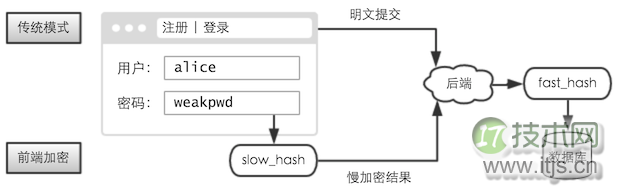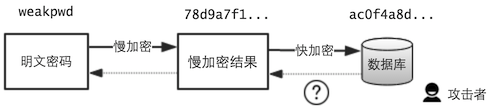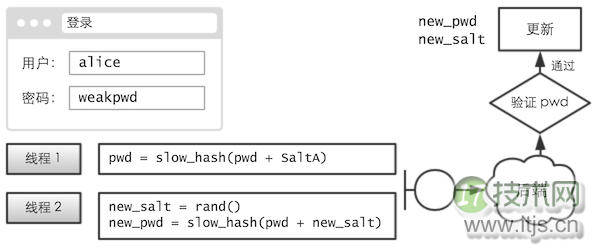﻿ 对抗拖库 —— Web 前端慢加密_模型算法_IT技术网 ﻿

# 对抗拖库 —— Web 前端慢加密

## 暴力破解（题图来自： netdna-ssl.com）

## 慢加密

```for i = 0 ~ 10000
x = md5(x)
end```

## 前端加密`back_fast_hash( front_slow_hash(password) )`

## 对抗预先计算

`front_slow_hash(password + username)`

```# 前端生成盐值
salt = rand()

# 提交时带上盐值## 强度策略

### 1.强度固定

`[前端] 完成 70% ----> [后端] 计算 30%`

### 2.强度可变

```# [注册阶段] 算力评估（线程 1 秒后中止）
while
x = hash(x)
step = step + 1
end```

```# [登录阶段] 先获得 step
for i = 0 ~ step
x = hash(x)
end```

### 4.异想天开方案## 0×08 性能优化

### 4.解释型

Alchemy 现在改名 FlasCC，还有开源版的 crossbridge

### 5.沙箱

`vector[k] = v`

## 0x0A 额外意义

### 3.增加撞库成本

「前端慢加密」需要消耗用户的计算力，这个缺点有时也是件好事。

## 0x0C 多线程慢加密

```for i = 0 ~ 10000
x = hash(x)
end```

```# 线程 1
for i = 0 ~ 2500
x1 = hash(x1)
end

# 线程 2
for i = 0 ~ 2500
x2 = hash(x2)
end

# ...```

﻿